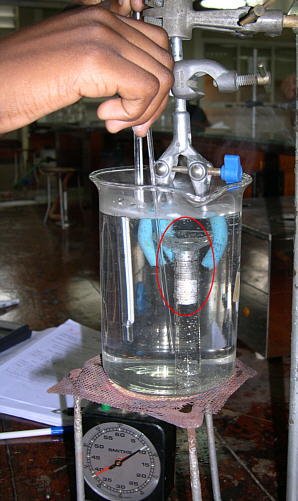### Experiment 24. Enthalpy of vaporisation of water from valour pressure measurement.

#### Objective

To determine the enthalpy of vaporisation of water from the measurement of vapour pressure at various temperatures.

#### Introduction

The Clausius - Clapeyron equation gives the relationship between the vapour pressure of a pure liquid and its temperature.
The relationship can be written simply as:
```
- Δ H vap
ln p =     ------------  + constant
R T
```
where Δ H is the enthalpy of vaporisation of the liquid.
The equation is derived from consideration that the Gibbs free energies for a liquid and vapour are equal when they are in equilibrium.
The other main assumptions are:
(i) the volume of vapour is assumed to be much greater than that of the liquid vapourised, and
(ii)it is assumed that the vapour behaves like an ideal gas.

In this experiment, a sample of air is trapped over water in an inverted measuring cylinder in a beaker. When the temperature of the apparatus is changed the number of moles of water vapour in the gas phase will vary according to the Clausius-Clapeyron equation, while that of air will remain constant. The number of moles of air in the mixture can be found by reducing the temperature of the whole apparatus to about 5 C. At that temperature it can be assumed that the vapour pressure of water is so small that the volume of gas measured corresponds only to the air present.

The enthalpy of vaporisation can then be calculated from a plot of ln p(H2O) (the vapour pressure) versus 1/T.

#### Apparatus Required:

10 cm3 (or 5 cm3) measuring cylinder, thermometer (preferably one reading to ~ 0.1 C), a tall beaker, bunsen burner.

#### Procedure(The values in parentheses refer to the case where a 5 cm3 measuring cylinder is used) Fill a 10-cm3 graduated cylinder about 80% full with distilled water. Cover the top with a finger and quickly invert and lower the cylinder into a tall beaker that has been filled with tap water. An air sample of 3 to 4 cm3 (1.5 to 2.0 cm3) should be trapped within the cylinder, record this volume and the temperature. Add more water if necessary to the beaker to ensure that the whole cylinder is surrounded by water and the bubble is completely immersed. Then heat with a Bunsen burner to approximately 80 C. During the heating, record the time, the volume and the temperature at every 5 C. When the volume of trapped gas expands beyond the scale on the cylinder, remove the burner and allow the water to cool slowly. When the gas begins to contract and the volume can be read again, record the volume and temperature to the closest 0.1 cm3 (0.05 cm3) and 0.1 C respectively. Stir the water bath frequently to avoid thermal gradients. As the water cools, make additional measurements of temperature at approximately 0.2 cm3 (0.1 cm3) intervals down to 50 C. You should be able to record at least 15 readings. After the temperature has reached 50 C, cool the water rapidly to less than 5 C by adding ice. Record the gas volume and the water temperature. Record the gas volume and the water temperatureagain at 5 and 10 mins after reaching ca 5 C. By then an equilibrium has been reached again. Obtain a value of the atmospheric pressure.

#### Calculations

1. Correct all volume readings by subtracting 0.2 cm3 (0.1 cm3) to compensate for the inverted meniscus. Using the measured values for volume and temperature from step 4 and the atmospheric pressure, calculate the number of moles n(air) of trapped air. Assume that the vapour pressure of water is negligible compared to atmospheric pressure at the low temperature.

2. For each temperature between 80 and 50 C calculate the partial pressure of air in the gas mixture.
```                                       n(air) RT
p(air) =    ------------
V
```
3. Calculate the vapour pressure of water at each temperature:
```
p (H2O) =   p (atm)  - p (air)
```
4. Plot ln p(H2O) versus 1/T and draw the best straight line.

5. Determine Δ H(vap) from the slope and p(H2O) at the temperature, X, given in class. Determine the standard deviation of Δ H(vap) by the "box method". See Appendix 7.

#### Discussion Questions

(i) Are there any assumptions other than those already mentioned which are worth considering?
(ii) Discuss the main sources of errors.Return to Chemistry, UWI-Mona, Home Page